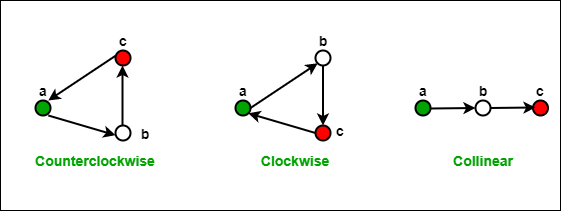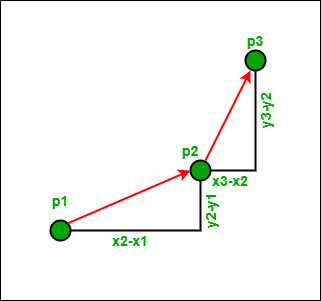# Orientation of 3 ordered points

• Difficulty Level : Easy
• Last Updated : 01 Sep, 2021

Orientation of an ordered triplet of points in the plane can be

• counterclockwise
• clockwise
• collinear

The following diagram shows different possible orientations of (a,b,c)

Attention reader! Don’t stop learning now. Get hold of all the important DSA concepts with the DSA Self Paced Course at a student-friendly price and become industry ready.  To complete your preparation from learning a language to DS Algo and many more,  please refer Complete Interview Preparation Course.

In case you wish to attend live classes with experts, please refer DSA Live Classes for Working Professionals and Competitive Programming Live for Students.If orientation of (p1, p2, p3) is collinear, then orientation of (p3, p2, p1) is also collinear.
If orientation of (p1, p2, p3) is clockwise, then orientation of (p3, p2, p1) is counterclockwise and vice versa is also true.
Example: Given three points p1, p2 and p3, find orientation of (p1, p2, p3).

```Input:   p1 = {0, 0}, p2 = {4, 4}, p3 = {1, 2}
Output:  CounterClockWise

Input:   p1 = {0, 0}, p2 = {4, 4}, p3 = {1, 1}
Output:  Collinear```

How to compute Orientation?

`The idea is to use slope.  ````Slope of line segment (p1, p2): σ = (y2 - y1)/(x2 - x1)
Slope of line segment (p2, p3): τ = (y3 - y2)/(x3 - x2)

If  σ > τ, the orientation is clockwise (right turn)

Using above values of σ and τ, we can conclude that,
the orientation depends on sign of  below expression:

(y2 - y1)*(x3 - x2) - (y3 - y2)*(x2 - x1)

Above expression is negative when σ < τ, i.e.,  counterclockwise```

Below is the implementation of above idea.

## C++

 `// A C++ program to find orientation of three points``#include ``using` `namespace` `std;` `struct` `Point``{``    ``int` `x, y;``};` `// To find orientation of ordered triplet (p1, p2, p3).``// The function returns following values``// 0 --> p, q and r are collinear``// 1 --> Clockwise``// 2 --> Counterclockwise``int` `orientation(Point p1, Point p2, Point p3)``{``    ``// See 10th slides from following link for derivation``    ``// of the formula``    ``int` `val = (p2.y - p1.y) * (p3.x - p2.x) -``              ``(p2.x - p1.x) * (p3.y - p2.y);` `    ``if` `(val == 0) ``return` `0;  ``// collinear` `    ``return` `(val > 0)? 1: 2; ``// clock or counterclock wise``}` `// Driver program to test above functions``int` `main()``{``    ``Point p1 = {0, 0}, p2 = {4, 4}, p3 = {1, 2};``    ``int` `o = orientation(p1, p2, p3);``    ``if` `(o==0)         cout << ``"Linear"``;``    ``else` `if` `(o == 1)  cout << ``"Clockwise"``;``    ``else`              `cout << ``"CounterClockwise"``;``    ``return` `0;``}`

## Java

 `// JAVA Code to find Orientation of 3``// ordered points``class` `Point``{``    ``int` `x, y;``    ``Point(``int` `x,``int` `y){``        ``this``.x=x;``        ``this``.y=y;``    ``}``}` `class` `GFG {``    ` `    ``// To find orientation of ordered triplet``    ``// (p1, p2, p3). The function returns``    ``// following values``    ``// 0 --> p, q and r are collinear``    ``// 1 --> Clockwise``    ``// 2 --> Counterclockwise``    ``public` `static` `int` `orientation(Point p1, Point p2,``                                         ``Point p3)``    ``{``        ``// See 10th slides from following link``        ``// for derivation of the formula``        ``int` `val = (p2.y - p1.y) * (p3.x - p2.x) -``                  ``(p2.x - p1.x) * (p3.y - p2.y);``     ` `        ``if` `(val == ``0``) ``return` `0``;  ``// collinear``     ` `        ``// clock or counterclock wise``        ``return` `(val > ``0``)? ``1``: ``2``;``    ``}``    ` `    ``/* Driver program to test above function */``    ``public` `static` `void` `main(String[] args)``    ``{``            ``Point p1 = ``new` `Point(``0``, ``0``);``            ``Point p2 = ``new` `Point(``4``, ``4``);``            ``Point p3 = ``new` `Point(``1``, ``2``);``            ` `            ``int` `o = orientation(p1, p2, p3);``            ` `            ``if` `(o==``0``)    ``            ``System.out.print(``"Linear"``);``            ``else` `if` `(o == ``1``) ``            ``System.out.print(``"Clockwise"``);``            ``else`             `            ``System.out.print(``"CounterClockwise"``);``        ` `    ``}``}` `//This code is contributed by Arnav Kr. Mandal.`

## Python3

 `# A Python3 program to find orientation of 3 points``class` `Point:``    ` `    ``# to store the x and y coordinates of a point``    ``def` `__init__(``self``, x, y):``        ``self``.x ``=` `x``        ``self``.y ``=` `y` `def` `orientation(p1, p2, p3):``    ` `    ``# to find the orientation of``    ``# an ordered triplet (p1,p2,p3)``    ``# function returns the following values:``    ``# 0 : Collinear points``    ``# 1 : Clockwise points``    ``# 2 : Counterclockwise``    ``val ``=` `(``float``(p2.y ``-` `p1.y) ``*` `(p3.x ``-` `p2.x)) ``-` `\``           ``(``float``(p2.x ``-` `p1.x) ``*` `(p3.y ``-` `p2.y))``    ``if` `(val > ``0``):``        ` `        ``# Clockwise orientation``        ``return` `1``    ``elif` `(val < ``0``):``        ` `        ``# Counterclockwise orientation``        ``return` `2``    ``else``:``        ` `        ``# Collinear orientation``        ``return` `0` `# Driver code``p1 ``=` `Point(``0``, ``0``)``p2 ``=` `Point(``4``, ``4``)``p3 ``=` `Point(``1``, ``2``)` `o ``=` `orientation(p1, p2, p3)` `if` `(o ``=``=` `0``):``    ``print``(``"Linear"``)``elif` `(o ``=``=` `1``):``    ``print``(``"Clockwise"``)``else``:``    ``print``(``"CounterClockwise"``)``    ` `# This code is contributed by Ansh Riyal`

## C#

 `// C# Code to find Orientation of 3``// ordered points``using` `System;``public` `class` `Point``{``    ``public` `int` `x, y;``    ``public` `Point(``int` `x,``int` `y)``    ``{``        ``this``.x = x;``        ``this``.y = y;``    ``}``}` `class` `GFG``{``    ` `    ``// To find orientation of ordered triplet``    ``// (p1, p2, p3). The function returns``    ``// following values``    ``// 0 --> p, q and r are collinear``    ``// 1 --> Clockwise``    ``// 2 --> Counterclockwise``    ``public` `static` `int` `orientation(Point p1, Point p2,``                                        ``Point p3)``    ``{``        ``// See 10th slides from following link``        ``// for derivation of the formula``        ``int` `val = (p2.y - p1.y) * (p3.x - p2.x) -``                ``(p2.x - p1.x) * (p3.y - p2.y);``    ` `        ``if` `(val == 0) ``return` `0; ``// collinear``    ` `        ``// clock or counterclock wise``        ``return` `(val > 0)? 1: 2;``    ``}``    ` `    ``/* Driver program to test above function */````    ``public` `static` `void` `Main(String[] args)``    ``{``            ``Point p1 = ``new` `Point(0, 0);``            ``Point p2 = ``new` `Point(4, 4);``            ``Point p3 = ``new` `Point(1, 2);``            ` `            ``int` `o = orientation(p1, p2, p3);``            ` `            ``if` `(o == 0)    ``                ``Console.WriteLine(``"Linear"``);``            ``else` `if` `(o == 1)``                ``Console.WriteLine(``"Clockwise"``);``            ``else`           `                ``Console.WriteLine(``"CounterClockwise"``);``        ` `    ``}``}` `/* This code contributed by PrinciRaj1992 */`

Output:

`CounterClockwise`

The concept of orientation is used in below articles:

Source: http://www.dcs.gla.ac.uk/~pat/52233/slides/Geometry1x1.pdf